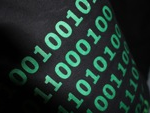0

## Power of 2

Published on Thursday, October 18, 2012 in , , , ,The recent Age Cards posts showed us one amazing application of the number 2 (base 2, in that case).

Today, we'll investigate some more amazing and surprising uses of the number 2, including some that can make you look like a genius!

For much of what you're going to learn here, you'll want to be comfortable with multiplying by 2 in your head, as well as the ability to easily divide by 2 mentally.

Incredibly, even if you can only multiply and divide by 2, you can still multiply any 2 numbers together! This is made possible by an unusual approach known as “Russian peasant multiplication” (sometimes also called “Egyptian multiplication”). It's explained in the following video:

Yes, this works with any 2 numbers, but depending on the numbers chosen, you may wind up adding more or fewer digits. If you're interested as to why this works, Dr. Math has some excellent explanations on this page, as well as this page.

Using a similar process, including the habit of dropping any decimals after dividing by 2, you can also quickly and easily convert any decimal number to a binary number. How? You take any given number, ask yourself whether it's odd or even. If it's odd, you're going to write down a 1, and if it's even, you'll write down a 0. You then divide by 2, and repeat this process. When you've completed this step all the way down to 1, you're done.

To make this clearer, let's try a small example, such as 19. Working this out step-by-step, we get this:

19 is odd: 1
(19/2 = 9.5, round down to get 9)
9 is odd: 1
(9/2 = 4.5, round down to get 4)
4 is even: 0
(4/2 = 2)
2 is even: 0
(2/1 = 1)
1 is odd: 1

Working from top to bottom, we write these numbers down from right to left (opposite of the way you would normally read) to get 10011. Double checking with Wolfram|Alpha, we see that 10011 is indeed the binary for 19.

As you can see, this is actually a bit simpler than the Russian peasant multiplication, but comes across as more impressive.

Let's try this with a higher number, such as 76, and simulate how you'd think and write it when showing this to someone else (the steps of dividing by 2 and rounding down are implied from one step to the next), noting that each step's result is written to the left of the previous result:

Think: 76 is even...
Write: 0

Think: 38 is even...
Write: 00

Think: 19 is odd...
Write: 100

Think: 9 is odd...
Write: 1100

Think: 4 is even...
Write: 01100

Think: 2 is even...
Write: 001100

Think: 1 is odd...
Write: 1001100

Yep, 1001100 is binary for 76! From the point of view of anybody watching, you seem to just work out the binary equivalent of the given number in your head without breaking a sweat!

Once you're comfortable converting decimal to binary, it's not hard to learn to work the other way.

To take a binary number and convert it, you're going to start from the leftmost 1. The leftmost binary digit will always be a 1 because, just like in decimal, you can put as many zeroes as you want to the left side without changing the number.

You start at the leftmost 1 with a mental total of 1. Every time you move to the right, regardless of the digit, you're always going to multiply by 2. If the digit you're at is a 1, then you're going to add a 1. Next, you move to the right again and multiply by 2, adding 1 if the digit is 1, and so on until you get to the rightmost digit.

Let's how this system is used to translate the binary number 11001. We start at the leftmost 1, with a mental total of 1:

11001
Mental Total: 1

(Move a digit to the right...)
11001
Mental Total: 1 * 2 = 2, 2 + 1 = 3 (the 1 is added because this digit is a 1)

(Move a digit to the right...)
11001
Mental Total: 3 * 2 = 6

(Move a digit to the right...)
11001
Mental Total: 6 * 2 = 12

(Move a digit to the right...)
11001
Mental Total: 12 * 2 = 24, 24 + 1 = 25 (the 1 is added because this digit is a 1)

Our final mental total is 25, so if we did this right, 11001 in binary should be 25 in decimal. A quick double-check with Wolfram|Alpha proves this is correct!

You can even work through this verbally quite easily, in this manner: “1...2.3...6...12...24.25!”

These binary and decimal conversions are derived from what's known in mathematics as Horner's method. This was originally developed as a way of evaluating polynomials, such as 3x2+2x+7 (Here's a quick tutorial on how to do that). However, since any number in any number base can be treated as a polynomial, such as 327 = (3 * 102) + (2 * 101) + (7 * 100), Horner's method also turns out to be useful for converting numbers from one base to another. You can learn the generalized method for converting any number in any base to decimal here.

Now you have 3 new amazing, and even amusing, abilities - Russian peasant multiplication, decimal-to-binary conversion, and binary-to-decimal conversion. Practice them, and you'll find them fun and maybe even useful!